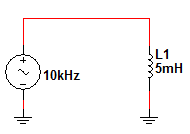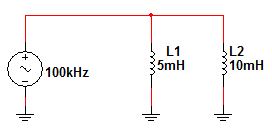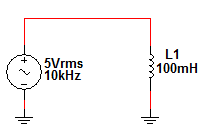# Inductors in AC and DC Circuits

Want create site? Find Free WordPress Themes and plugins.

The main action of an inductor is to resist a change in current.  However, since the current in a DC circuit is constant, there is no induced voltage developed instantaneously across the inductor.  The inductor does resist the initial inrush of current based on the time constant of the circuit. In an AC circuit, the current is constantly reversing itself; so, inductors have a major impact on the circuit.

## RL Time Constant

Energy is stored in the inductor when current is applied.  The rate at which this energy is stored is dependent upon the time constant (τ) of the circuit.  The time constant is a ratio of the inductance of a circuit to its resistance.

$\tau =\frac{L}{R}$

The time constant is in seconds when the inductance and resistance are in henries and ohms, respectively.  Just as in a capacitor, it takes five-time constants for the inductor to reach full storage.  It also takes five-time constants to return to zero from full storage.

## Inductors in a DC Circuit Example

If a series RL circuit has a resistance of 1 kΩ and an inductance of 1.0 mH, determine the time constant of the circuit.

$\tau =\frac{L}{R}=\frac{1mH}{1k\Omega }=1~\mu s$

Increasing and Decreasing Current

Inductors inherently resist a change in current, so full current cannot pass through it instantaneously.  The current through the inductor increases exponentially with the time constant when a voltage is applied.  Table 1 shows the approximate percentages of current through the inductor at each time constant value.  After five time constants, the full current of the circuit is passing through the inductor.  At this point, the inductor appears as a short to the DC voltage source.

Table 1. Table of Increasing Current Percentages

 Number of Time Constants Approximate % of Final Current 1 63 2 86 3 95 4 98 5 99 (considered 100%)

Since the inductor resists a change in current, the current decreases exponentially with the same time constant when the voltage is removed.  Table 2 shows the approximate percentages of current through the inductor at each time constant value.  After the five-time constants have passed, the current through the inductor has returned to zero.

Table 2 – Table of Decreasing Current Percentages

 Number of Time Constants Approximate % of Initial Current 1 37 2 14 3 5 4 2 5 1 (considered 0%)

## Inductors in a DC Circuit Example 2

Using Figure 1, determine the current through L1 after two-time constants.Figure 1 – Example RL Current Circuit

First, we must find the time constant.

$\tau =\frac{L}{R}=\frac{10mH}{1.2k\Omega }=8.33\mu s$

Now, calculate the final current (once the inductor has become a short).

${{I}_{F}}=\frac{{{V}_{T}}}{R}=\frac{12V}{1.2k\Omega }=10mA$

Now, simply use the time constant percentage found in Table 1 to find the current.

${{I}_{at~2\tau }}={{I}_{F}}\times 0.86=10mA\times 0.86=8.6mA$

## Inductive Reactance

The amount of opposition an inductor creates in an AC circuit is dependent upon the inductance value and the frequency of the voltage source.  The inductive reactance (XL) of a circuit is a measure of this opposition.

${{X}_{L}}=2\pi fL$

XL is inductive reactance (Ω),

f is frequency (Hz)

L is inductance (F)

Inductive reactance is given in ohms when frequency and inductance are in hertz and henries, respectively.  As you can see from the formula for inductive reactance, it is directly proportional to the frequency of the source and the inductance value of the inductor.

## Inductors in an AC Circuit Example 1

Determine the inductive reactance in Figure 2.Figure 2 – Example Inductive Reactance Circuit

${{X}_{L}}=2\pi fL=2\pi \times 10kHz\times 5mH$

${{X}_{L}}=314.2\Omega$

When inductors are combined in series or parallel circuits, the total inductive reactance is found in much the same way as the total inductance.  Since the inductive reactance is dependent upon the total inductance, the formula looks the same for the total reactance.  In a series circuit, the individual reactances add up to form the total inductive reactance.

${{X}_{L\left( total \right)}}={{X}_{L1}}+{{X}_{L2}}+{{X}_{L3}}+\ldots$

In a parallel circuit, the total inductance is found by finding the reciprocal of the sum of the reciprocals of the individual inductances.  The total inductive reactance is found the same way.

${{X}_{L\left( total \right)}}=\frac{1}{\frac{1}{{{X}_{L1}}}+\frac{1}{{{X}_{L2}}}+\frac{1}{{{X}_{L3}}}+\ldots }$

## Inductors in an AC Circuit Example 2

Determine the total inductive reactance of the circuit in Figure 3.Figure 3– Example Parallel Inductive Reactance Circuit

First, we must find the individual reactances.

${{X}_{L1}}=2\pi fL=2\pi \times 100kHz\times 5mH=3.142k\Omega$

${{X}_{L2}}=2\pi fL=2\pi \times 100kHz\times 10mH=6.283k\Omega$

Now, substitute the individual reactances into the appropriate total reactance formula.

${{X}_{L\left( total \right)}}=\frac{1}{\frac{1}{{{X}_{L1}}}+\frac{1}{{{X}_{L2}}}}=\frac{1}{\frac{1}{3.142k\Omega }+\frac{1}{6.283k\Omega }}=2.095k\Omega$

Since inductive reactance is a measure of the opposition to current flow in an inductor, it is similar to a resistance measurement.  You can use the total inductive reactance in a purely inductive circuit to determine the total current using Ohm’s Law.  Simply substitute XL for R, and solve as usual.

${{I}_{T}}=\frac{{{V}_{T}}}{{{X}_{L}}}$

In an AC circuit, however, both current and voltage must be expressed either in peak or root-mean-square (rms) values for the formulas to work.

## Inductors in an AC Circuit Example 3

Find the rms current in Figure 4.Figure 4 – Example AC Ohm’s Law Circuit

First, find the inductive reactance.

${{X}_{L}}=2\pi fL=2\pi \times 10kHz\times 100mH=6.283k\Omega$

Now, we can substitute the values into Ohm’s Law to find the rms current in the circuit.

${{I}_{rms}}=\frac{{{V}_{rms}}}{{{X}_{L}}}=\frac{5V}{6.283k\Omega }=796\mu A$

Did you find apk for android? You can find new Free Android Games and apps.